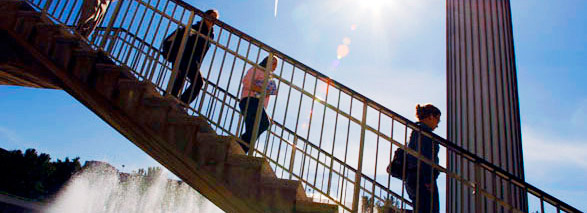## Geometry and Topology Department of Mathematics and Statistics

The geometry/topology group at the University at Albany conducts research in a variety of areas, e.g., geometric topology, geometric group theory, group actions on manifolds, equivariant homotopy theory, and topological data analysis. The interests of some group members are detailed below.

### Justin Curry"I work in the area of applied topology, which has emerged in recent decades out of the interaction of topology, which provides a rigorous notion of "shape," and problems arising naturally from data science, engineering, and physics. Much of my work concerns theoretical foundations for topological data analysis (TDA) and persistent homology, with an eye towards future applications. My mathematical expertise lies primarily in sheaf theory, stratification theory, category theory, representation theory, posets, analysis and some elementary combinatorics. My applied interests are relatively broad, but recently are concerned with time series and image analysis."

### Boris Goldfarb"I work in the area of topology that studies manifolds and spaces with manifold strata. These are the most common and useful topological spaces. Surgery theory is a program that proposes how to classify such spaces. This classification amounts to answering questions in homotopy theory and algebraic K-theory.

"I am especially fascinated by the relations of this subject with geometric group theory, equivariant homotopy theory, coarse geometry, and by how recent breakthroughs in these subjects can be exploited in classification of manifolds. I also work on applications of algebraic topology to manifold learning and other questions in data analytics."

### Michael Lesnick"Michael Lesnick's research focuses primarily on topological data analysis (TDA). He works on the theoretical foundations of the subject, and also on the applied and computational aspects. Recently, he has been especially interested in the design of practical software tools for TDA and in applications to biology. Michael completed his Ph.D. in Computational Mathematics at Stanford in 2012. Before coming to Albany, he was a postdoc at the Institute for Advanced Study, the Institute for Mathematics and its Applications, and Princeton University."

### Marco Varisco"My research is in algebraic and geometric topology. The main motivations come from studying and trying to classify manifolds and their symmetries. For manifolds of sufficiently high dimension, such questions can be attacked using algebraic invariants, like for example the algebraic K-theory groups of group algebras. The structure of these groups is predicted by the far-reaching Farrell--Jones conjecture in algebraic K-theory. My own work focuses on this conjecture and uses methods from stable and equivariant homotopy theory and geometric group theory. Together with my colleague Alex Tchernev, we also apply similar methods to algebraic problems in commutative algebra and algebraic geometry."

### Matthew Zaremsky"My research interests lie in the field of geometric group theory, which means that I use topological and geometric tools to understand properties of infinite groups. Some topics that particularly interest me include classifying spaces, finiteness properties, Bieri-Neumann-Strebel-Renz invariants, homological stability, and topology at infinity. One of the topological tools I use frequently is discrete Morse theory, which is a way of leveraging "local" data about a space to make "global" conclusions, both about the space itself and about any group acting nicely on it. Some examples of groups that I study include matrix groups, braid groups, right-angled Artin groups, groups acting on trees and other CAT(0) spaces, groups of automorphisms of free groups, and the extended family of Thompson's groups."